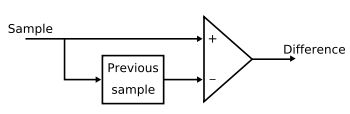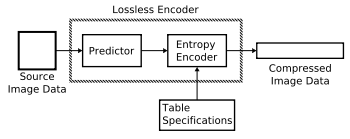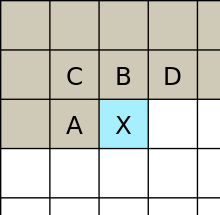# Lossless JPEG

Lossless JPEG is a 1993 addition to JPEG standard by the Joint Photographic Experts Group to enable lossless compression. However, it might be used as an umbrella term to refer to all lossless compression schemes developed by the Joint Photographic Expert group. They include JPEG 2000 and JPEG-LS.Figure 1: DPCM encoder modelFigure 2: Simplified block diagrams for lossless modeLossless JPEG was developed as a late addition to JPEG in 1993, using a completely different technique from the lossy JPEG standard. It uses a predictive scheme based on the three nearest (causal) neighbors (upper, left, and upper-left), and entropy coding is used on the prediction error. The standard Independent JPEG Group libraries cannot encode or decode it, but Ken Murchison of Oceana Matrix Ltd. wrote a patch that extends the IJG library to handle Lossless JPEG. Lossless JPEG has some popularity in medical imaging, and is used in DNG and some digital cameras to compress raw images, but otherwise was never widely adopted.

## Lossless mode of operation

Lossless JPEG is actually a mode of operation of JPEG. This mode exists because the discrete cosine transform (DCT) based form cannot guarantee that encoder input would exactly match decoder output. Unlike the lossy mode which is based on the DCT, the lossless coding process employs a simple predictive coding model called differential pulse code modulation (DPCM). This is a model in which predictions of the sample values are estimated from the neighboring samples that are already coded in the image. Most predictors take the average of the samples immediately above and to the left of the target sample. DPCM encodes the differences between the predicted samples instead of encoding each sample independently. The differences from one sample to the next are usually close to zero. A typical DPCM encoder is displayed in Fig.1. The block in the figure acts as a storage of the current sample which will later be a previous sample.

The main steps of lossless operation mode are depicted in Fig.2. In the process, the predictor combines up to three neighboring samples at A, B, and C shown in Fig.3 in order to produce a prediction of the sample value at the position labeled by X. The three neighboring samples must be already encoded samples. Any one of the predictors shown in the table below can be used to estimate the sample located at X. Any one of the eight predictors listed in the table can be used. Note that selections 1, 2, and 3 are one-dimensional predictors and selections 4, 5, 6, and 7 are two-dimensional predictors. The first selection value in the table, zero, is only used for differential coding in the hierarchical mode of operation. Once all the samples are predicted, the differences between the samples can be obtained and entropy-coded in a lossless fashion using Huffman coding or arithmetic coding.

Selection-value Prediction
0 No prediction
1 A
2 B
3 C
4 A + B – C
5 A + (B – C)/2
6 B + (A – C)/2
7 (A + B)/2

Typically, compressions using lossless operation mode can achieve around 2:1 compression ratio for color images. This mode is quite popular in the medical imaging field, and defined as an option in DNG standard, but otherwise it is not very widely used because of complexity of doing arithmetics on 10, 12 or 14bpp values on typical embedded 32bit processor and a little resulting gain in space.

## JPEG-LS

JPEG-LS is a lossless/near-lossless compression standard for continuous-tone images. Its official designation is ISO-14495-1/ITU-T.87. It is a simple and efficient baseline algorithm which consists of two independent and distinct stages called modeling and encoding. JPEG-LS was developed with the aim of providing a low-complexity lossless and near-lossless image compression standard that could offer better compression efficiency than lossless JPEG. It was developed because at the time, the Huffman coding-based JPEG lossless standard and other standards were limited in their compression performance. Total decorrelation cannot be achieved by first order entropy of the prediction residuals employed by these inferior standards. JPEG-LS, on the other hand, can obtain good decorrelation. Part 1 of this standard was finalized in 1999. Part 2, released in 2003, introduced extensions such as arithmetic coding. The core of JPEG-LS is based on the LOCO-I algorithm, that relies on prediction, residual modeling and context-based coding of the residuals. Most of the low complexity of this technique comes from the assumption that prediction residuals follow a two-sided geometric distribution (also called a discrete Laplace distribution) and from the use of Golomb-like codes, which are known to be approximately optimal for geometric distributions. Besides lossless compression, JPEG-LS also provides a lossy mode ("near-lossless") where the maximum absolute error can be controlled by the encoder. Compression for JPEG-LS is generally much faster than JPEG 2000 and much better than the original lossless JPEG standard.

### LOCO-I algorithm

Prior to encoding, there are two essential steps to be done in the modeling stage: decorrelation (prediction) and error modeling.

#### Decorrelation/prediction

In the LOCO-I algorithm, primitive edge detection of horizontal or vertical edges is achieved by examining the neighboring pixels of the current pixel X as illustrated in Fig.3. The pixel labeled by B is used in the case of a vertical edge while the pixel located at A is used in the case of a horizontal edge. This simple predictor is called the Median Edge Detection (MED) predictor or LOCO-I predictor. The pixel X is predicted by the LOCO-I predictor according to the following guesses:The three simple predictors are selected according to the following conditions: (1) it tends to pick B in cases where a vertical edge exists left of the X, (2) A in cases of an horizontal edge above X, or (3) A + B – C if no edge is detected.

#### Context modeling

The JPEG-LS algorithm estimates the conditional expectations of the prediction errorsusing corresponding sample meanswithin each context Ctx. The purpose of context modeling is that the higher order structures like texture patterns and local activity of the image can be exploited by context modeling of the prediction error. Contexts are determined by obtaining the differences of the neighboring samples which represents the local gradient:The local gradient reflects the level of activities such as smoothness and edginess of the neighboring samples. Notice that these differences are closely related to the statistical behavior of prediction errors. Each one of the differences found in the above equation is then quantized into roughly equiprobable and connected regions. For JPEG-LS, the differences g1, g2, and g3 are quantized into 9 regions and the region are indexed from −4 to 4. The purpose of the quantization is to maximize the mutual information between the current sample value and its context such that the high-order dependencies can be captured. One can obtain the contexts based on the assumption thatAfter merging contexts of both positive and negative signs, the total number of contexts iscontexts. A bias estimation could be obtained by dividing cumulative prediction errors within each context by a count of context occurrences. In LOCO-I algorithm, this procedure is modified and improved such that the number of subtractions and additions are reduced. The division-free bias computation procedure is demonstrated in . Prediction refinement can then be done by applying these estimates in a feedback mechanism which eliminates prediction biases in different contexts.

#### Coding corrected prediction residuals

In the regular mode of JPEG-LS, the standard uses Golomb–Rice codes which are a way to encode non-negative run lengths. Its special case with the optimal encoding value 2k allows simpler encoding procedures.

#### Run length coding in uniform areas

Since Golomb–Rice codes are quite inefficient for encoding low entropy distributions because the coding rate is at least one bit per symbol, significant redundancy may be produced because the smooth regions in an image can be encoded at less than 1 bit per symbol. To avoid having excess code length over the entropy, one can use alphabet extension which codes blocks of symbols instead of coding individual symbols. This spreads out the excess coding length over many symbols. This is the “run” mode of JPEG-LS and it is executed once a flat or smooth context region characterized by zero gradients is detected. A run of west symbol “a” is expected and the end of run occurs when a new symbol occurs or the end of line is reached. The total run of length is encoded and the encoder would return to the “regular” mode.

## JPEG 2000

Main article: JPEG 2000

JPEG 2000 includes a lossless mode based on a special integer wavelet filter (biorthogonal 3/5). JPEG 2000's lossless mode runs more slowly and has often worse compression ratios than JPEG-LS on artificial and compound images. JPEG 2000 fares better than the UBC implementation of JPEG-LS on digital camera pictures. JPEG 2000 is also scalable, progressive, and more widely implemented.

## References

1. Pennebaker, W. B. & Mitchell, J. L. (1993). JPEG Still Image Data Compression Standard. New York: Van Nostrand Reinhold. ISBN 0-442-01272-1.
2. ITU-T. ISO DIS 10918-1 Digital compression and coding of continuous-tone still images (JPEG). Recommendation T.81.
3. Wallace, C. K. (1991). "The JPEG still picture compression standard". Communications of the ACM. 34 (4): 31–44. doi:10.1109/30.125072.
4. "HP Labs LOCO-I/JPEG-LS Home Page". Hewlett-Packard Development Company. May 13, 2005. Retrieved 24 October 2011.
5. "T.87 : Information technology – Lossless and near-lossless compression of continuous-tone still images – Baseline". International Telecommunication Union. Retrieved 24 October 2011.
6. M. J. Weinberger, G. Seroussi, and G. Sapiro, “LOCO-I: A low complexity, context-based, lossless image compression algorithm,” in Proc. 1996 Data Compression Conference, Snowbird, UT, Mar. 1996, pp. 140–149.
7. M. Weinberger, G. Seroussi, and G. Sapiro, “The LOCO-I lossless image compression algorithm: Principles and standardization into JPEG-LS,” IEEE Trans. Image Processing, vol. 9, no. 8, pp. 1309–1324, Aug. 2000, originally as Hewlett-Packard Laboratories Technical Report No. HPL-98-193R1, November 1998, revised October 1999. Available from .
8. http://www.hpl.hp.com/loco/HPL-98-193R1.pdf LOCO-I algorithm
9. Memon, Nasir D.; Wu, Xiaolin; Sippy, V. & Miller, G. (1997). "Interband coding extension of the new lossless JPEG standard". Proc. SPIE Int. Soc. Opt. Eng. 3024 (47): 47–58. Bibcode:1997SPIE.3024...47M. doi:10.1117/12.263270.
10. http://www.jpeg.org/public/wg1n1816.pdf
11. http://itohws03.ee.noda.sut.ac.jp/~matsuda/mrp/
12. http://www.imagecompression.info/gralic/LPCB.html